## Monday, August 27, 2018

### Unizor - Physics4Teens - Mechanics - Rotational Dynamics - Problems

Notes to a video lecture on http://www.unizor.com

Problems on
Rotational Dynamics

Problem 1

Consider a device that consists of a wheel of radius r and mass M, freely rotating on some fixed axis, a thread rolled around it a few times and a point-object of mass m hanging off that thread.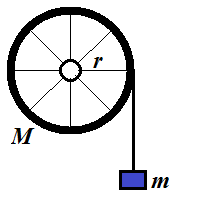Assume that all mass of a wheel is concentrated in its rim, while spokes are weightless.

There is no friction in the rotating wheel.

A thread is weightless and unstrechable.

The force of gravity pulls the object down with free fall acceleration g.

What will be the acceleration of an object?

Solution

The weight of a wheel is balanced by the reaction of fixed axis and, therefore, can be ignored.

The only force acting tangentially on a wheel is the tension of a tread T trying to rotate it.

Therefore, we can equate its momentum τ=T·r (torque) to a product of its moment of inertia I and angular acceleration α:

τ = T·r = I·α

The fact that all mass of a wheel is concentrated in its rim allows us
to easily calculate its moment of inertia. We can imagine a rim
consisting of a large number N of point-objects of mass M/N each. The moment of inertia of each is (M/N)·r² and combined moment of inertia of a wheel is N·(M/N)·r²=M·r². If the mass is not concentrated in the rim, analogous logic would lead us to more involved calculations of inertial mass.

Considering, I=M·r² and r·α=a (where a is a linear acceleration of a thread) we can write the following equation:

T·r = M·r²·a/r = M·r·a

Radius cancels out and we get

T = M·a

Notice that this is exactly the same equation as the Newton's Second Law, as if an object of mass M is pulled along a straight line by a force T with linear acceleration a.

Another important detail is that this formula is independent of a radius
of a wheel - a direct consequence of the fact that the mass of a wheel
is concentrated in its rim.

Analyzing the movement of an object hanging on a thread, we conclude that it moves with linear acceleration a down by the forces of gravity (directed down) and tension of a thread (directed up). Therefore, using the Newton's Second Law,

m·g − T = m·a

Now we have a system of two equations with two unknowns T and a, which is easy to solve.

Substitute T from the first equation to the second:

m·g − M·a = m·a

m·g = (M + m) · a

a = m·g / (M + m)

Problem 2

Calculate a moment of inertia of disk of mass M and radius R rotating around an axis going through its center perpendicularly to its surface.

Solution

Divide a disk into a set of concentric rings of infinitesimal width dr. Let the inner radius of a particular ring be r and it outer radius be r+dr.

Moment of inertia of each ring is

I(r) = m·r²

where m is its mass (see the previous Problem 1 for explanation).

Mass of a ring m is a mass of a disk M multiplied by a ratio of a ring's area 2πr·dr to the area of an entire disk πR².

So, the moment of inertia of our ring of radius r and infinitesimal width dr is

I(r) = [M·2πr·dr/(πR²)]·r²

Integrating this from r=0 to r=R, we get

Idisk(M, R) =

=
[M·2πr·dr/(πR2)]·r2 =

= (2M/R2)r3
dr =

= (2M/R2)·(r4/4) =

= M·R2/2

Problem 3

Consider Problem 1, but change a wheel with all mass concentrated in the
rim with a wheel with mass evenly distributed inside the circumference,
making it a disk.

Solution

We follow the same logic as in Problem 1.

The weight of a wheel is balanced by the reaction of fixed axis and, therefore, can be ignored.

The only force acting tangentially on a wheel is the tension of a tread T trying to rotate it.

Therefore, we can equate its momentum τ=T·r (torque) to a product of its moment of inertia I and angular acceleration α:

τ = T·r = I·α

The fact that all mass of a wheel is evenly distributed within its
circumference allows us to easily calculate its moment of inertia using
the Problem 2 above:
I=M·r²/2
Linear acceleration a and angular acceleration α are related:

r·α=a

Therefore,

T·r = [M·r²/2]·(a/r) = M·r·a/2

Radius cancels out and we get

T = M·a/2

An important detail is that this formula is independent of a radius of a wheel.

Analyzing the movement of an object hanging on a thread, we conclude that it moves with linear acceleration a down by the forces of gravity (directed down) and tension of a thread (directed up). Therefore, using the Newton's Second Law,

m·g − T = m·a

Now we have a system of two equations with two unknowns T and a, which is easy to solve.

Substitute T from the first equation to the second:

m·g − M·a/2 = m·a

m·g = (m + M/2) · a

a = m·g / (m + M/2)

If we compare this formula with the one in Problem 1, we see that final acceleration is greater because denominator is smaller.

So, the disk in this problem will rotate faster than a wheel with empty middle part from Problem 1.

Problem 4

Let's rotate a small ball of mass M within a horizontal plane on a thread of length D. The thread will make certain angle φ with the horizon. Experiments show that the angle will be smaller if a ball rotates faster.

Determine the relationship between angular speed of rotation ω, mass of a ball M, length of a thread D and angle φ, ignoring friction and air resistance.

Solution

The tension of a thread T keeps a ball on its orbit. Radius of an orbit is R=D·cos(φ).

The tension of a thread T serves dual purpose - its vertical component T·sin(φ) acts against gravity M·g, its horizontal component T·cos(φ) acts as a centripetal force and should be equal to M·V2/R, where V is a linear speed of a ball, which is equal, in turn, to R·ω, where ω is angular speed of rotation of a ball.

So, we have the following equations:
T·sin(φ) = M·g

T·cos(φ) = M·(V2)/R = M·R·ω2 = M·D·cos(φ)·ω2

The second equation is simplified to
T = M·D·ω2

Substituting it to the first equation,
D·ω2·sin(φ) = g

Therefore,
sin(φ) = g/[D·ω2]

For a given angular speed we can find an angle of a thread to horizon φ. It in inversely proportional to a length of a thread D and to a square of angular speed ω, which seems to be reasonable.

Interestingly, this angle is independent of the mass M.

## Tuesday, August 21, 2018

### Unizor - Physics4Teens - Mechanics - Dynamics - Angular Momentum

Notes to a video lecture on http://www.unizor.com

Angular Momentum

Recall the rotational equivalent of the Newton's Second Law:

τ = I·α

where

τ = F·r - a torque, a product of tangentially applied force F and the distance from the point of application of force to the axis of rotation r;

I = m·R² - a moment of inertia, a product of inertial mass of a point-object by a square of its distance from the axis of rotation R (might be different from the distance r above);

α - angular acceleration of rotation.

It is very important to correspond straight line translational elements of motion with their rotational counterparts:

 Translation Rotation ForceF Torqueτ = F·r Linear Accelerationa Angular Accelerationα = a/r InertialMassm Momentof InertiaI = m·r² Newton'sSecond LawF=m·a RotationalEquivalentτ = I·α

Now we will consider a rotational equivalent of a familiar equation
between impulse and momentum in translational motion along a straight
line.

As is known from the previous material, there is a correspondence between these quantities: impulse of the force F exhorted during an infinitesimal time period dt equals to an increment of the momentum d(m·v), where m is mass and v - velocity of an object:

dt = d(m·v)

Let's see if the corresponding rotational characteristics of motion have similar dependency.

We know the rotational equivalent of the Newton's Second Law τ=I·α.

Multiplying it by dt, we obtain

τ·dt = I·α·dt

Since a product of angular acceleration α and infinitesimal time interval dt is an infinitesimal increment of angular speed dω, there is an equality

τ·dt = I·dω = d(I·ω)

The latter fully corresponds to an equation between impulse and momentum
of translational movement. It represents the relationship between rotational impulse τ·dt of torque τ during time interval dt and increment of rotational (angular) momentum d(I·ω) of an object with a moment of inertia I and angular speed ω.

We can continue our table of correspondence between translational motion
along a straight line and rotation along a circular trajectory.

 Translation Rotation ImpulseF·dt Rotational Impulseτ·dt Momentumd(m·v)=F·dt Rotational Momentumd(I·ω)=τ·dt

Trivial consequence of an equation

τ·dt = d(I·ω)

is that

τ = d(I·ω)/dt = (I·ω)'

From this follows that if the balance of all torques acting on an object is zero, the rotational momentum remains constant.

This is the Law of Conservation of the rotational (angular) momentum.

## Monday, August 20, 2018

### Unizor - Physics4Teens - Mechanics - Dynamics - Moment of Inertia

Notes to a video lecture on http://www.unizor.com

Moment of Inertia

Recall the rotational equivalent of the Newton's Second Law:

τ = I·α

where

τ = F·r - a torque, a product of tangentially applied force F and the distance from the point of application of force to the axis of rotation r;

I = m·R² - a moment of inertia, a product of inertial mass of a point-object by a square of its distance from the axis of rotation R (might be different from the distance r above);

α - angular acceleration of rotation.

It is very important to correspond straight line translational elements of motion with their rotational counterparts:

 Translation Rotation ForceF Torqueτ = F·r Linear Accelerationa Angular Accelerationα = a/r InertialMassm Momentof InertiaI = m·r² Newton'sSecond LawF=m·a RotationalEquivalentτ = I·α

In this lecture we will study the moment of inertia and its properties. We will also calculate the moment of inertia in a few simple cases.

The first very important property of the moment of inertia is that it is additive.
It means that the moment of inertia of two rigidly connected
point-objects rotating around the same axis of rotation equals to sum of
their individual moments of inertia.

Let's explain why.

Consider a rigid weightless disk rotating around an axis going through
its center perpendicularly to it and two point-objects of mass m1 and m2 fixed on its surface at distances, correspondingly, r1 and r2.

Assume that this disk with both fixed on it objects is rotating with angular acceleration α caused by some force F acting on a distance R from its center tangentially to a circle of a radius R within a plane of this disk.

The picture below illustrates this.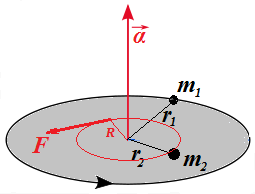Our purpose is to explain that the combined moment of inertia of both objects equals to a sum of their corresponding individual moments of inertia.

The fact that object of mass m1 on a distance r1 from the axis of rotation has angular acceleration α implies that there is a force f1 acting on it tangentially to its trajectory such that (from rotational equivalent of the Newton's Second Law):

f1·r1 = I1·α

where I1=m1·r1² - moment of inertia of this object.

Similarly, the fact that object of mass m2 on a distance r2 from the axis of rotation has angular acceleration α implies that there is a force f2 acting on it tangentially to its trajectory such that (from rotational equivalent of the Newton's Second Law):

f2·r2 = I2·α

where I2=m2·r2² - moment of inertia of this object.

Behavior of this system depends only on parameters specified above -
masses, distances from the center of a disk and forces. So, if we change
the initial position of masses without changing their distances from
the center, the system will work exactly the same.

Our first modification then will be to shift the position of mass m2 along its circular trajectory of radius r2 to a position, where it is on the same radius as m1. So, both masses and a center of a disk are on one line.

Similarly, let's shift the point of application of force F to a point on the same line where our two objects are now located without changing the distance R
from the point of application of the force to a center. Now both masses
and a point of application of main force that rotates the disk are on
the same line with a center of the disk, while their distances from a
center are still the same as before.

As we know, the angular acceleration of a rotating object depends on its
mass, its distance from an axis of rotation and a torque of a force
rotating this object.

If force f1 applied directly to an object of mass m1 located on a distance r1 from the axis of rotation caused the angular acceleration α, any other force having the same torque τ1=f1·r1 would result in the same angular acceleration.

Let's replace then the force f1 acting on a distance r1 from the axis with force g1=f1·r1/R, acting on a distance R at the point where our main force F was applied. There will be no change in angular acceleration since the torque acting on our object is the same:

τ'1 = (f1·r1/R)·R = f1·r1 = τ1

Same trick with the second object results in using force g2=f2·r2/R, acting on a distance R at the point where our main force F was applied, with no change in the behavior of the system.

τ'2 = (f2·r2/R)·R = f2·r2 = τ2

We have brought all forces (g1, g2 and F)
to the same point of application. They act in the same direction -
tangentially to a circular trajectory. We know that the effect of main
force F applied to this point is equivalent to a combined effect of forces g1=f1·r1/R and g2=f2·r2/R applied to the same point in the same direction. Therefore, sum of forces g1 and g2 must be equal to force F:

g1 + g2 = F

Now what remains is simple algebra.

f1·r1/R + f2·r2/R = F

f1·r1 + f2·r2 = F·R

τ1 + τ2 = F·R

I1·α + I2·α = F·R

(I1 + I2)·α = F·R

The expression on the right is the torque of the main force F acting tangentially to a circle of rotation on a distance R from the axis:

τ = F·R

Therefore, the expression on the left, according to rotational equivalent of the Newton's Second Law, must be a moment of inertia of the entire system I multiplied by the system's angular acceleration α:

I·α = τ = F·R

from which follows the equality

I·α = (I1 + I2)·α

and, finally,

I = I1 + I2

That means that the combined moment of inertia of two objects equals to a sum of their individual moments of inertia.

In short, moment of inertia is additive.

The most important consequence of this is that, to calculate a moment of
inertia of a rotating solid object of some complicated geometric shape,
we can divide it in small (strictly speaking, infinitesimally small)
pieces, calculate a moment of inertia of each piece and add all these
moments of inertia together (strictly speaking, integrating them) to get
a moment of inertia of the whole rotating solid object.

Let's calculate a moment of inertia of some solids, using this approach.

1. Rod rotating around its edge

Consider a solid thin rod of a length L and mass m rotating around an axis that is perpendicular to it and going through its one end.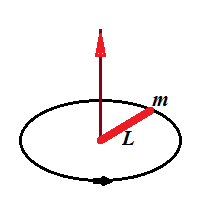To calculate its moment of inertia, we divide it in small pieces of the length dr, located on a distance r from the axis and having mass dm=(m/L)·dr.

Each such piece has a moment of inertia

dI = (dm)·r² = (m/L)·r²·dr

Since moment of inertia is additive, all we have to do now is to integrate this by r from 0 to L.

The indefinite integral of function is r³/3. So, by Newton-Leibniz formula the result of integration on an interval [0,L] is

L³/3 − 0³/3 = L³/3.

So, the total moment of inertia of a rod is

Irod = (m/L)·L³/3 = m·L²/3

2. Rod rotating around its center

Let's calculate the moment of inertia, if the rod rotates around its center point.

We can consider now this rod as a system of two small half-rods, each being a half of the original one, with length L/2 and mass m/2.

Each half-rod rotates around its end, so the formula above is applicable to each half-rod.

Its moment of inertia is

Ihalf = (m/2)·(L/2)²/3 = m·L²/24

The whole rod, being a system of two half-rods, has a momentum of
inertia equal to a sum of momentums of its two halves, so its value is
double of the above moment of inertia for each half-rod:

Iwhole = m·L²/12

## Wednesday, August 15, 2018

### Unizor - Physics4Teens - Mechanics - Rotational Dynamics

Notes to a video lecture on http://www.unizor.com

Torque

So far we were mostly considering translational motion of point-objects - a motion along a straight line with or without external forces
acting upon this object. We have specified three Newton's Laws of this
motion and derived a lot of interesting facts based on these laws.

Let's recall these laws.

The Newton's First Law is the familiar Law of Inertia that states that
an object at rest stays at rest and an object in uniform motion stays in
this uniform motion, unless acted upon by unbalanced forces.

The Newton's Second Law brings quantitative relationship to vector of force (F), mass (m) and vector of acceleration (a):

F = m·a

The Newton's Third Law states that for every action there is an equal in magnitude and oppositely directed reaction.

Rotational motion obeys the rules in many respects analogous to the laws of translational motion, except we have to change linear movement to rotation, which, in essence, is an angular movement with constant radius.

Consider a point-object of some mass m connected by a weightless rigid rod of the length r to an axis, around which this object can rotate within a plane of rotation that is perpendicular to an axis of rotation.

The picture below illustrates such a movement and also indicates the position of angular velocity ω
of a rotation, which was explained earlier, when we studied the
Kinematics of rotation (we assume your familiarity with this topic and
strongly recommend to refresh it before proceeding any further).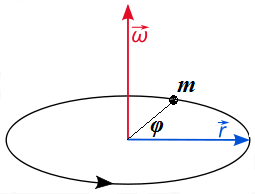Let's discuss the similarities and differences between translational movement along a straight line and rotation around an axis within a plane of rotation perpendicular to this axis.

Obviously, the time concept remains the same in both types of motion.

There is a clear rotational analogy to the Newton's First Law.

An object at rest stays at rest and an object in uniform rotation
stays in this uniform rotation, unless acted upon by unbalanced forces.

The Newton's Third Law is not really specific for a form of motion, so there is no need to address it separately at this moment.

The Newton's Second Law requires certain modification to be applied to rotation.

Let's address the main concept of Dynamics - the force - in connection to rotation.

The very important characteristic of force in Dynamics of translational movement along a straight line is that it can be measured by its effect on objects of certain inertial mass. Thus, a measure of force that gives linear acceleration of 1 m/sec² to an object of inertial mass of 1 kg is 1 newton. The same force applied to an object of 0.5 kg of inertial mass will cause 2 m/sec² linear acceleration.

The same force applied to different objects of the same inertial mass will cause the same linear acceleration etc.

The situation with rotational movement is not the same.

Consider a simple experiment of opening a door. If you apply a force to
open a door at its edge opposite to hinges, where the handle is usually
located, it opens relatively faster than if you apply exactly the same
force in the middle of a door. The closer a point of application of the
same force to hinges - the slower a door opens, if the force applied to
it is the same. In an extreme case, when we apply the pressure where the
hinges are, a door will not open at all.

As a continuation of this experiment, if we want to achieve the same
speed of the opening of a door by applying the force at different
distances from the hinges, we need more efforts for a point of force
application located closer to the hinges.

We can measure the force, the distance from the hinges of a point of application of this force and an angular acceleration of the door and experimentally come up with the fact that for the fixed force the angular acceleration of the door is proportional to the distance of a point of application of the force from the hinges.

Moreover, leaving the point of application of the force the same and changing the force, we can determine that angular acceleration is proportional to the force.

What it means is that in a case of rotation a force by itself does not
determine the final motion of a rotating object. It's a product of a
force F and radius to a point this force is applied r, that determines the final effect. This product τ = F·r is called the torque and it is rotational equivalent of a force in translational movement.

 Translation Rotation ForceF Torqueτ = F·r

Returning to a picture above, we can apply some force F to
any point on the rigid rod, connecting our point-object to an axis of
rotation, directing this force perpendicularly to the rod, and observe
that the resulting angular acceleration of the object is proportional to
both the force F and the distance r from the axis to a point of application of this force, thus proportional to torque τ = F·r.

Now we have concluded that an angular acceleration α of rotational motion is proportional to a torque τ. This is analogous to linear acceleration a of translational motion being proportional to a force F. The coefficient of proportionality for translational motion is inertial mass m of an object (this is the Newton's Second Law F=m·a).

The obvious question is, what is the coefficient of proportionality between angular acceleration and torque?

Answer to this question will result in rotational equivalent of the Newton's Second Law.

We have experimentally established that equal torques produce equal angular accelerations.

Consider a rotation illustrated on the picture above. Assume that the point of application of force F is exactly at the point-object of mass m rotating around an axis at a distance r
from it on a rigid weightless rod. Assume further that our force acts
within a plane of rotation and directed perpendicularly to the rod.

During an infinitesimal time interval dt the motion of an object can be considered as linear and, therefore, the Newton's Second Law can be applied, giving F=m·a.

Now we can express it in terms of angular acceleration and torque as follows:

a = r·α

τ = F·r

Hence,

F·r = (m·a)·r = m·r²·α

Finally,

τ = (m·r²)·α

One more logical step is needed. We started from a force applied on an object itself at a distance r
from an axis. But we have experimentally established that equal torques
produce equal actions. It means that some other force applied to some
other point will produce the same effect, causing the same angular
acceleration, as long as the torque is the same.

So, the equality τ=(m·r²)·α is universal, regardless of point of application of force since it depends not on force, but on torque.

The above equality represents the rotational analogue of the Newton's Second Law.

Some generalization can be applied to the above.

What if the force, acting within a plane of rotation, is not perpendicular to a rod?

Obvious solution is to replace vector F
with its projection onto a line on the rotation plane that is
perpendicular to a radius and to multiply the product of two scalars F·r by a sine of an angle between corresponding vectors, effectively using a vector product Fr.

So, more general definition of a torque is a vector (or, more precisely, pseudo-vector)

τ = Fr

whose direction, like a direction of an angular acceleration, is along an axis of rotation.

So, for rotational movement the vectors of torque τ and angular acceleration α are collinear, similarly to collinearity of vectors of force F and linear acceleration a for translational movement.

We'd like to note that for purposes of simplicity in this course we will
rarely deal with forces not perpendicular to a radius of rotation.

IMPORTANT TERMINOLOGY POINTS

1. The torque τ is often called the moment of force.

2. Recall the expression tying together a torque τ and an angular acceleration α

τ = (m·r²)·α

The expression m·r² is called moment of inertia, is symbolized by letter I, which allows to specify the formula above as

τ = I·α

where I=m·r² is a moment of inertia, playing the same role in this equation as inertial mass m in the Newton's Second Law and representing resistance to a rotational force.

 Translation Rotation ForceF Torqueτ = F·r Accelerationa Angular Acc.α = a/r InertialMassm Momentof InertiaI = m·r² Newton'sSecond LawF=m· RotationalEquivalentτ = I·α

## Monday, August 13, 2018

### Unizor - Physics4Teens - Mechanics - Rotational Kinematics

Notes to a video lecture on http://www.unizor.com

Rotational Kinematics

So far we were mostly considering translational motion of point-objects - a motion along a straight line with or without external forces acting upon this object.

Rotational motion obeys the rules in many respects analogous to the laws of translational motion, except we have to change linear movement to rotation.

Consider a point-object m connected by a rigid rod of the length r to an axis, around which this object can rotate within a plane of rotation that is perpendicular to an axis of rotation.

The picture below illustrates such a movement and also indicates the position of angular velocity ω of a rotation, which we will explain later.Let's discuss the similarities and differences between translational movement along a straight line and rotation around an axis within a plane perpendicular to this axis (a plane of rotation).

The first main concept of translational motion is position or distance from the beginning of motion (for a straight line movement) as a function of time. In rotational motion its equivalent is angle of rotation from some original position as a function of time.

 Translation Rotation Distances(t) Angleφ(t)

The next concept is speed or (better) velocity of translational motion. This is a first derivative of position (or distance) by time:

v(t) = s'(t).

Its equivalent for rotational motion is angular speed, which is a first derivative of angle of rotation by time:

ω(t) = φ'(t)

While vector character of speed of translational motion is obvious and is reflected in the term velocity, vector character of angular speed is less obvious.

The angle of rotation from the first glance is a scalar function of time. But only from the first glance.

In theory, rotational motion always assumes existence of an axis of
rotation and a plane of rotation. To reflect these characteristics and a
magnitude of angular speed, an angular speed is represented by a vector
from a center of rotation along an axis of rotation perpendicularly to a
plane of rotation with a magnitude equal to a value of angular speed.

This allows to represent the rotation in its full spectrum of
characteristics - magnitude, axis, plane of rotation. The picture above
represents angular speed as a vector ω(t), which we may call angular velocity vector.

There is one more characteristic of rotational motion not yet discussed - its direction. It is also reflected in angular velocity
as a vector by its direction. In theory, we can choose two different
directions along the axis of rotation. The direction chosen is such
that, if we look from its end onto a plane of rotation, the rotation is
counterclockwise. Another interpretation of this is the "rule of the
right hand" because if you put you right hand on a plane of rotation
such that your finger go around the axis of rotation pointing to a
direction of rotation, your thumb points to a direction of the angular velocity vector.

So, angular velocity vector represents axis, plane, direction of rotation as well as magnitude of angular speed.

To be more precise, since this vector representation of angular velocity is a little unusual, it is customary to call it "pseudo-vector" instead of "vector".

During infinitesimal time interval dt an object rotating around an axis on a radius r turns by an angle dφ(t), covering the distance ds(t)=r·dφ(t) (this is the length of an arc of radius r and angle , according to a known formula of geometry).

From this follows:

ds(t)/dt = r·dφ(t)/dt or

v(t) = r·ω(t)

 Translation Rotation Speedv(t)=s'(t)v(t)=r·ω(t) Angular Speedω(t)=φ'(t)ω(t)=v(t)/r

The next concept is acceleration that needs its rotational analogue. Obviously, it's the first derivative of angular velocity or the second derivative of an angle of rotation by time.

Using the vector interpretation of angular velocity, we can consider angular acceleration as a vector as well. It is also directed along the axis of rotation.

During infinitesimal time interval dt an angular velocity ω(t) changes by dω(t).

From this follows relationship between linear acceleration a and angular acceleration α:

a(t) = dv(t)/dt = r·dω(t)/dt or

a(t) = r·α(t)

 Translation Rotation Accelerationa(t)=v'(t)a(t)=r·α(t) Angular Acc.α(t)=ω'(t)α(t)=a(t)/r

Obviously, integrating the definitions of angular velocity ω and angular acceleration α for constant angular acceleration, we come up with formulas similar to those familiar from translational movement:

ω(t) = ω(0) + α·t

φ(t) = φ(0) + ω(0)·t + α·t²/2

Angular acceleration as a vector (as a pseudo-vector, to be exact), is colinear to the axis of rotation, because angular velocity is.

If rotation goes as on the above picture and the speed of rotation increases, the angular acceleration would be directed upwards, the same way as the angular velocity.

## Monday, August 6, 2018

### Unizor - Physics4Teens - Mechanics - Statics - Equilibrium

Notes to a video lecture on http://www.unizor.com

Equilibrium

Statics is a part of Mechanics that studies forces not as
quantitative measure of a motion they cause (that is a subject of
Dynamics), but from the more fundamental viewpoint of whether these
forces are or are not balanced, that might or might not cause the motion
of objects these forces act upon.

Historically, studies of static aspects of forces precede quantitative
studies of motion in Dynamics. People, first of all, were concerned with
how to build bridges and buildings, so that they stay in place and not
destroyed by gravity, wind or load. And a concept of equilibrium is a central point of Statics, it's goal and purpose.

Equilibrium, as it is understood in Statics, is a state of forces, that result in an object acted upon to be in the state of rest.

A person standing on a floor is at rest because two main forces that act
upon him, the gravity and the reaction of the floor are equal in
magnitude and opposite in direction, they balance each other, which
results in a state of equilibrium.

A person standing on the weights to check his weight is in a state of equilibrium
because its weight is balanced by elastic force inside the weights that
is equal in magnitude and opposite in direction to the force of
gravity. The equality in magnitude allows to measure the weight by
measuring the elasticity inside the weights.

A building is in a state of equilibrium because its each part's weight is balanced by equal in magnitude and opposite in direction force of reaction.

An airplane, flying horizontally on its route, is in a state of vertical equilibrium
because its weight is balanced by the lifting power of the air under
its wings, where the air pressure is larger because of the wings' shape.

Let's study forces applied to the same object and balance each other causing this object to be in equilibrium.

As we know, force is a vector. All forces acting on the same point-object can be added as vectors, using the rules of the Vector Algebra.

If the result is a null-vector, we have an equilibrium.

So, if there are N forces Fi (where 1 ≤ i ≤ N) acting simultaneously on the same point-object, the condition of equilibrium is

ΣFi = 0 .

Let's consider a few examples.

1. An object of weight W is hanging on three threads as pictured below.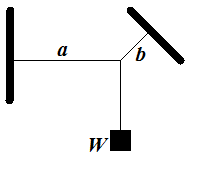Thread a is horizontal, thread b is at angle φ to horizon.

The system is in equilibrium.

What is the magnitude of tension forces Ta and Tb on threads a and b?

Solution

There are three forces acting at the point where all threads are connected:

vertical down - the weight W of the object;

tension Ta horizontally to the left along the a thread;

tension Tb at angle φ to horizon along the b thread.

Since the system is in equilibrium, this point where threads come
together does not move in any direction. In particular, it does not move
in a vertical, nor horizontal direction.

This is sufficient to determine magnitude of tension vectors Ta and Tb.

In the horizontal direction the force of gravity is irrelevant, so the
only two forces acting on out object in horizontal direction are tension
Ta acting to the left and a projection of tension Tb on the horizontal line acting to the right.

That gives the first equation about magnitudes of tension forces:

Ta = Tb·cos(φ)

Now consider the vertical direction. The thread a is irrelevant. So, the only two forces acting vertically, are weight W pulling down and a vertical component of Tb that is equal to Tb·sin(φ) pulling upwards.

This gives the second equation

Tb·sin(φ) = W

From these equations we derive:
Tb = W/sin(φ)

After which we can find Ta:

Ta = W·cos(φ)/sin(φ)

2. Two objects of mass M (larger, on an inclined plane) and m (smaller, hanging freely over the edge of an inclined plane) are connected with a thread that goes over a pulley.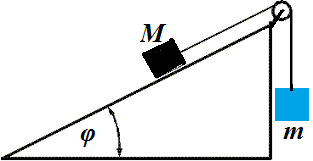What is the angle of an inclined plane φ for this system to be in equilibrium?

Ignore the friction.

Solution

Let's represent the weight of an object on an inclined plane as a sum of two forces:

one is perpendicular to the plane and balanced by a plane's reaction;

another is parallel to the plane and balanced by a tension of a thread.

To be in equilibrium, the force of weight of an object hanging freely
over the edge of an inclined plane must be equal in magnitude to a